# Programming a two-body problem simulator in JavaScript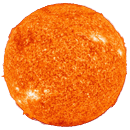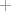Mass ratio: 0.10
Eccentricity: 0.10

In this tutorial we will program a simulation of motion of two celestial bodies in HTML/JavaScript. This work is mostly based on what I learned from Dr Rosemary Mardling, who is an astrophysicist at Monash University. In addition, this code uses techniques we developed in harmonic oscillator tutorial, which would be a good starting place for those who are new to this topic. Please feel free to use the full source code of this simulation for any purpose.

## Our approach

We have already done a simulation of motion of two bodies in the Earth orbit simulator tutorial. There we derived equations of motion from the Lagrangian and used them to move the planet around the Sun. For simplicity, we choose the center of mass of two bodies to be at the center of the Sun, which stayed stationary at the origin of our coordinate system. Here we will use a different approach and allow two bodies to move around a common center of mass.

## Our coordinate system

A surprising fact about the motion of two bodies is that their common center of mass remains still (or moves at constant velocity). We can take advantage of this fact and place the origin of the coordinate system at the center of mass (Fig. 1).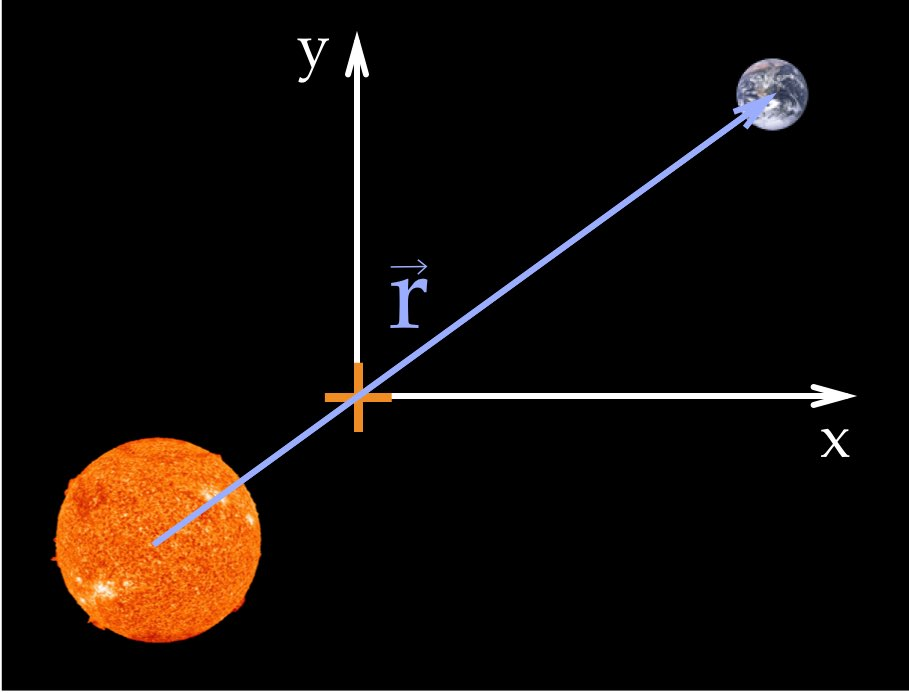Figure 1: A coordinate system with the origin at the common center of mass of the two bodies.

## Newton’s law of gravitation

The main equation used in our simulation is the Newton’s law of universal gravitation (Eq. 1). It says that the force of gravitational attraction between two bodies is proportional to the product of their masses and inversely proportional to the square of the distance between them.(1)

## Equation of motion

After a series of algebraic manipulations and removing dimensions, we can turn Eq. 1 into an equation of motion of two bodies shown in Eq. 2. Here vector r describes the position of second body relative to the first one. This vector is shown as blue arrow on Fig. 1.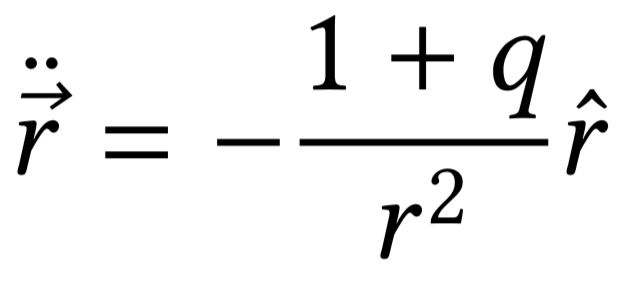(2)

The variable q in Eq. 2 is the mass ratio of the bodies (i.e. mass of the Earth divided by Sun’s mass). The two dots above vector r mean the second time derivative.

## Equations of motion for x and y

Next, we write Eq. 2 in terms of x and y coordinates, which gives a system of two second-order non-linear differential equations: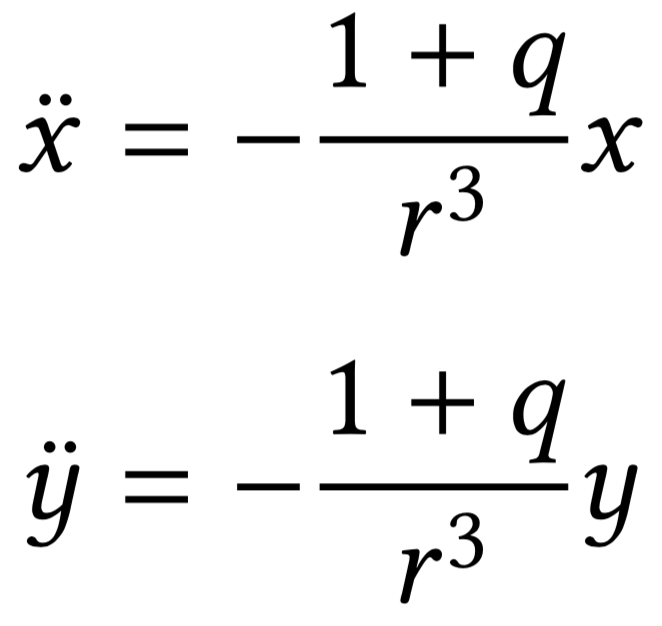(3)

## Reducing the order of ODEs

We will solve Eq. 3 numerically. In order to do this, we need to translate it into a system of first-order ODEs by making the following substitutions: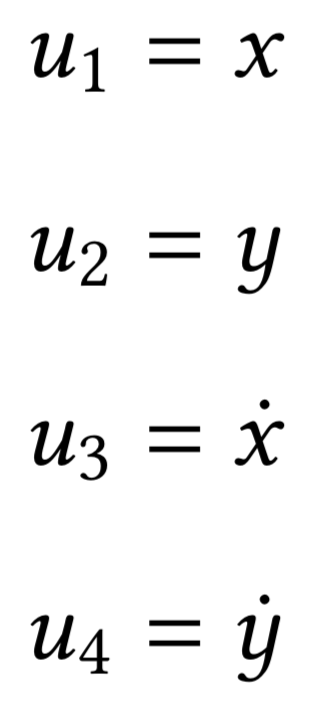(4)

We will store the values of these four variables in the `state.u` array of the `physics` object:

``````var state = {
// Four variables used in the differential equations
// First two elements are x and y positions, and second two are x and y components of velocity
u: [0, 0, 0, 0]
}
``````

Using the new variables we can now translate two second-order differential equations (Eq. 4) into four first-order ones: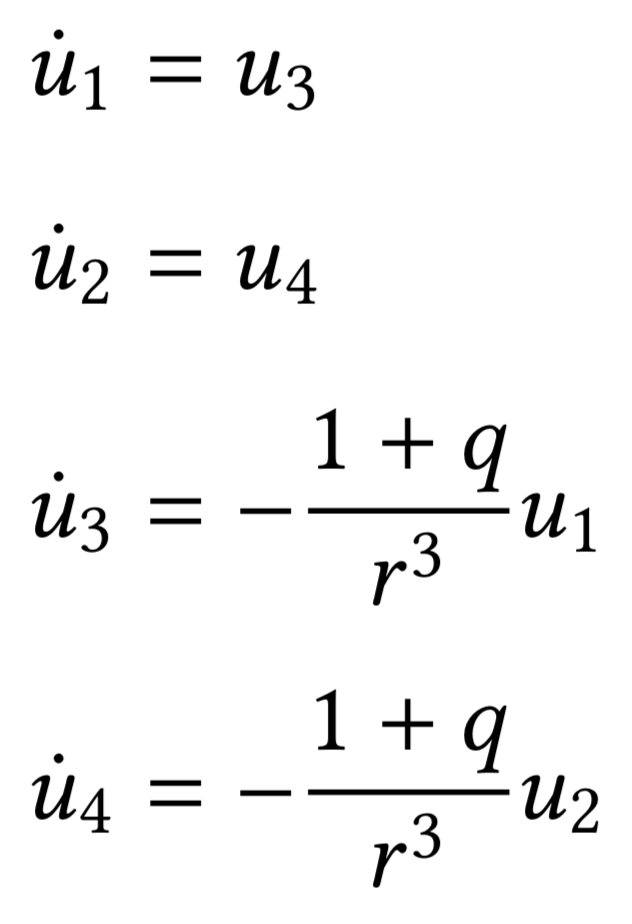(5)

We can now write a function that returns the derivatives of the four variables:

``````// Calculate the derivatives of the system of ODEs that describe equation of motion of two bodies
function derivative() {
var du = new Array(state.u.length);

// x and y coordinates
var r = state.u.slice(0,2);

// Distance between bodies
var rr = Math.sqrt( Math.pow(r,2) + Math.pow(r,2) );

for (var i = 0; i < 2; i++) {
du[i] = state.u[i + 2];
du[i + 2] = -(1 + state.masses.q) * r[i] / (Math.pow(rr,3));
}

return du;
}
``````

## Solve ODEs numerically

Now we can use a numerical method to solve the ODEs. In Earth orbit simulator we used Euler’s method, but here we will try another popular method called Runge-Kutta:

``````var timestep = 0.15;
rungeKutta.calculate(timestep, state.u, derivative);
``````

We will run this code at each frame of the animation and it will save the new values of positions and velocities in `state.u`. The first two elements of this array are the x and y positions of the r vector from Fig. 1.

## Drawing two bodies on the screen

We are almost done. We have found the vector r, which describes the position of Earth relative to the Sun. We will now use this vector to calculate the positions of two bodies on screen. The trick here is to use the mass-distance relation shown in Fig. 2.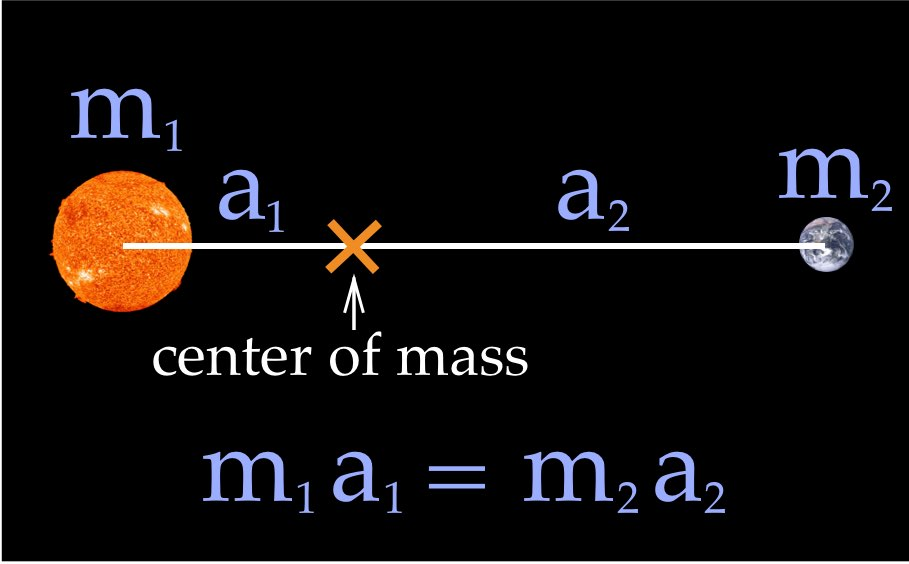Figure 2: A relation between masses of two bodies and distances to the common center of mass.

With this knowledge, we can finally calculate the positions of the two bodies. The positions are saved in the `state.positions` array and then used to show the two bodies on screen.

``````function calculateNewPosition() {
r = 1; // Distance between two bodies
// m12 is the sum of two massses
var a1 = (state.masses.m2 / state.masses.m12) * r;
var a2 = (state.masses.m1 / state.masses.m12) * r;

state.positions.x = -a2 * state.u;
state.positions.y = -a2 * state.u;

state.positions.x = a1 * state.u;
state.positions.y = a1 * state.u;
}
``````

## Credits

1. This work is based on code and lectures by Dr Rosemary Mardling from Monash University.

2. “The Blue Marble” image: NASA/Apollo 17 crew; taken by either Harrison Schmitt or Ron Evans, source, source.

3. “The Sun photographed at 304 angstroms” image: NASA/SDO (AIA), source, source.